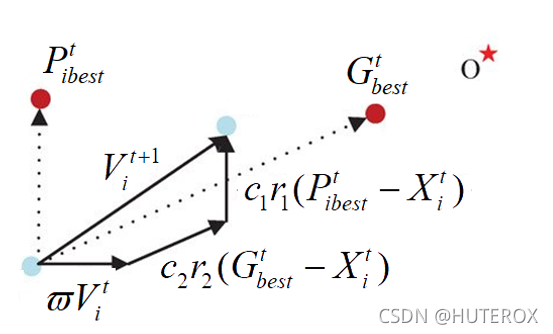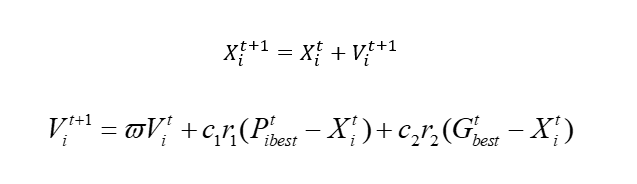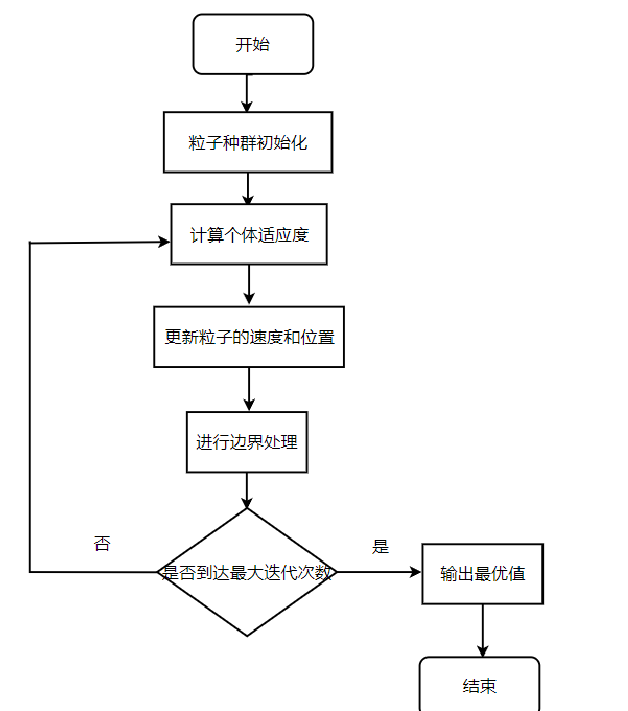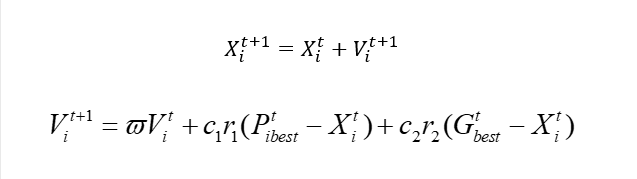# PSO算法PSO算法通过模拟鸟类迁移来实现咱们的优化，这个怎么来的，就不说了，就说说这个核心。

## 算法流程PSO算法没有交叉和变异运算，依靠粒子速度完成搜索，并且在迭代进化中只有最优的粒子把信息传递给其它粒子，搜索速度快。

PSO算法具有记忆性，粒子群体的历史最好位置可以记忆并传递给其它粒子。

## 简单实现

ok,我们来看一下最简单的实现：

``````import numpy as np
import random

class PSO_model:
def __init__(self,w,c1,c2,r1,r2,N,D,M):
self.w = w # 惯性权值
self.c1=c1
self.c2=c2
self.r1=r1
self.r2=r2
self.N=N # 初始化种群数量个数
self.D=D # 搜索空间维度
self.M=M # 迭代的最大次数
self.x=np.zeros((self.N,self.D))  #粒子的初始位置
self.v=np.zeros((self.N,self.D))  #粒子的初始速度
self.pbest=np.zeros((self.N,self.D))  #个体最优值初始化
self.gbest=np.zeros((1,self.D))  #种群最优值
self.p_fit=np.zeros(self.N)
self.fit=1e8 #初始化全局最优适应度

# 目标函数，也是适应度函数（求最小化问题）
def function(self,x):
A = 10
x1=x
x2=x
Z = 2 * A + x1 ** 2 - A * np.cos(2 * np.pi * x1) + x2 ** 2 - A * np.cos(2 * np.pi * x2)
return Z

# 初始化种群
def init_pop(self):
for i in range(self.N):
for j in range(self.D):
self.x[i][j] = random.random()
self.v[i][j] = random.random()
self.pbest[i] = self.x[i] # 初始化个体的最优值
aim=self.function(self.x[i]) # 计算个体的适应度值
self.p_fit[i]=aim # 初始化个体的最优位置
if aim < self.fit:  # 对个体适应度进行比较，计算出最优的种群适应度
self.fit = aim
self.gbest = self.x[i]

# 更新粒子的位置与速度
def update(self):
for t in range(self.M): # 在迭代次数M内进行循环
for i in range(self.N): # 对所有种群进行一次循环
aim=self.function(self.x[i]) # 计算一次目标函数的适应度
if aim<self.p_fit[i]: # 比较适应度大小，将小的负值给个体最优
self.p_fit[i]=aim
self.pbest[i]=self.x[i]
if self.p_fit[i]<self.fit: # 如果是个体最优再将和全体最优进行对比
self.gbest=self.x[i]
self.fit = self.p_fit[i]
for i in range(self.N): # 更新粒子的速度和位置
self.v[i]=self.w*self.v[i]+self.c1*self.r1*(self.pbest[i]-self.x[i])+ self.c2*self.r2*(self.gbest-self.x[i])
self.x[i]=self.x[i]+self.v[i]
print("最优值：",self.fit,"位置为：",self.gbest)

if __name__ == '__main__':
# w,c1,c2,r1,r2,N,D,M参数初始化
w=random.random()
c1=c2=2#一般设置为2
r1=0.7
r2=0.5
N=30
D=2
M=200
pso_object=PSO_model(w,c1,c2,r1,r2,N,D,M)#设置初始权值
pso_object.init_pop()
pso_object.update()

``````

# 解决TSP

## 数据表示

``````      # 群体的初始化和路径的初始化
self.population = np.array( * self.num_pop * self.num).reshape(
self.num_pop, self.num)
self.fitness =  * self.num_pop

"""
计算城市的距离，我们用矩阵表示城市间的距离
"""
self.__matrix_distance = self.__matrix_dis()
``````

## 区别``````
def cross_1(self, path, best_path):
r1 = np.random.randint(self.num)
r2 = np.random.randint(self.num)
while r2 == r1:
r2 = np.random.randint(self.num)

left, right = min(r1, r2), max(r1, r2)
cross = best_path[left:right + 1]
for i in range(right - left + 1):
for k in range(self.num):
if path[k] == cross[i]:
path[k:self.num - 1] = path[k + 1:self.num]
path[-1] = 0
path[self.num - right + left - 1:self.num] = cross
return path

``````

``````    def mutation(self,path):
r1 = np.random.randint(self.num)
r2 = np.random.randint(self.num)
while r2 == r1:
r2 = np.random.randint(self.num)
path[r1],path[r2] = path[r2],path[r1]
return path

``````

## 完整代码

ok,现在我们来看到完整的代码：

``````import numpy as np
import matplotlib.pyplot as plt

class HybridPsoTSP(object):
def __init__(self ,data ,num_pop=200):
self.num_pop = num_pop  # 群体个数
self.data = data        # 城市坐标
self.num =len(data)     # 城市个数

# 群体的初始化和路径的初始化
self.population = np.array( * self.num_pop * self.num).reshape(
self.num_pop, self.num)
self.fitness =  * self.num_pop

"""
计算城市的距离，我们用矩阵表示城市间的距离
"""
self.__matrix_distance = self.__matrix_dis()

def __matrix_dis(self):
"""
计算14个城市的距离，将这些距离用矩阵存起来
:return:
"""
res = np.zeros((self.num, self.num))
for i in range(self.num):
for j in range(i + 1, self.num):
res[i, j] = np.linalg.norm(self.data[i, :] - self.data[j, :])
res[j, i] = res[i, j]
return res

def cross_1(self, path, best_path):
r1 = np.random.randint(self.num)
r2 = np.random.randint(self.num)
while r2 == r1:
r2 = np.random.randint(self.num)

left, right = min(r1, r2), max(r1, r2)
cross = best_path[left:right + 1]
for i in range(right - left + 1):
for k in range(self.num):
if path[k] == cross[i]:
path[k:self.num - 1] = path[k + 1:self.num]
path[-1] = 0
path[self.num - right + left - 1:self.num] = cross
return path

def mutation(self,path):
r1 = np.random.randint(self.num)
r2 = np.random.randint(self.num)
while r2 == r1:
r2 = np.random.randint(self.num)
path[r1],path[r2] = path[r2],path[r1]
return path

def comp_fit(self, one_path):
"""
计算，咱们这个路径的长度，例如A-B-C-D
:param one_path:
:return:
"""
res = 0
for i in range(self.num - 1):
res += self.__matrix_distance[one_path[i], one_path[i + 1]]
res += self.__matrix_distance[one_path[-1], one_path]
return res

def out_path(self, one_path):
"""
输出我们的路径顺序
:param one_path:
:return:
"""
res = str(one_path + 1) + '-->'
for i in range(1, self.num):
res += str(one_path[i] + 1) + '-->'
res += str(one_path + 1) + '\n'
print(res)

def init_population(self):
"""
初始化种群
:return:
"""
rand_ch = np.array(range(self.num))
for i in range(self.num_pop):
np.random.shuffle(rand_ch)
self.population[i, :] = rand_ch
self.fitness[i] = self.comp_fit(rand_ch)

def main(data, max_n=200, num_pop=200):
Path_short = HybridPsoTSP(data, num_pop=num_pop)  # 混合粒子群算法类
Path_short.init_population()  # 初始化种群

# 初始化路径绘图
fig, ax = plt.subplots()
x = data[:, 0]
y = data[:, 1]
ax.scatter(x, y, linewidths=0.1)
for i, txt in enumerate(range(1, len(data) + 1)):
ax.annotate(txt, (x[i], y[i]))
res0 = Path_short.population
x0 = x[res0]
y0 = y[res0]
for i in range(len(data) - 1):
plt.quiver(x0[i], y0[i], x0[i + 1] - x0[i], y0[i + 1] - y0[i], color='r', width=0.005, angles='xy', scale=1,
scale_units='xy')
plt.quiver(x0[-1], y0[-1], x0 - x0[-1], y0 - y0[-1], color='r', width=0.005, angles='xy', scale=1,
scale_units='xy')
plt.show()
print('初始染色体的路程: ' + str(Path_short.fitness))

# 存储个体极值的路径和距离
best_P_population = Path_short.population.copy()
best_P_fit = Path_short.fitness.copy()

min_index = np.argmin(Path_short.fitness)

# 存储当前种群极值的路径和距离
best_G_population = Path_short.population[min_index, :]
best_G_fit = Path_short.fitness[min_index]

# 存储每一步迭代后的最优路径和距离
best_population = [best_G_population]
best_fit = [best_G_fit]

# 复制当前群体进行交叉变异
x_new = Path_short.population.copy()

for i in range(max_n):
# 更新当前的个体极值
for j in range(num_pop):
if Path_short.fitness[j] < best_P_fit[j]:
best_P_fit[j] = Path_short.fitness[j]
best_P_population[j, :] = Path_short.population[j, :]

# 更新当前种群的群体极值
min_index = np.argmin(Path_short.fitness)
best_G_population = Path_short.population[min_index, :]
best_G_fit = Path_short.fitness[min_index]

# 更新每一步迭代后的全局最优路径和解
if best_G_fit < best_fit[-1]:
best_fit.append(best_G_fit)
best_population.append(best_G_population)
else:
best_fit.append(best_fit[-1])
best_population.append(best_population[-1])

# 将每个个体与个体极值和当前的群体极值进行交叉
for j in range(num_pop):
# 与个体极值交叉
x_new[j, :] = Path_short.cross_1(x_new[j, :], best_P_population[j, :])
fit = Path_short.comp_fit(x_new[j, :])
# 判断是否保留
if fit < Path_short.fitness[j]:
Path_short.population[j, :] = x_new[j, :]
Path_short.fitness[j] = fit
# 与当前极值交叉
x_new[j, :] = Path_short.cross_1(x_new[j, :], best_G_population)
fit = Path_short.comp_fit(x_new[j, :])
if fit < Path_short.fitness[j]:
Path_short.population[j, :] = x_new[j, :]
Path_short.fitness[j] = fit
# 变异
x_new[j, :] = Path_short.mutation(x_new[j, :])
fit = Path_short.comp_fit(x_new[j, :])
if fit <= Path_short.fitness[j]:
Path_short.population[j] = x_new[j, :]
Path_short.fitness[j] = fit

if (i + 1) % 20 == 0:
print('第' + str(i + 1) + '步后的最短的路程: ' + str(Path_short.fitness[min_index]))
print('第' + str(i + 1) + '步后的最优路径:')
Path_short.out_path(Path_short.population[min_index, :])  # 显示每一步的最优路径

Path_short.best_population = best_population
Path_short.best_fit = best_fit
return Path_short  # 返回结果类

if __name__ == '__main__':
data = np.array([16.47, 96.10, 16.47, 94.44, 20.09, 92.54,
22.39, 93.37, 25.23, 97.24, 22.00, 96.05, 20.47, 97.02,
17.20, 96.29, 16.30, 97.38, 14.05, 98.12, 16.53, 97.38,
21.52, 95.59, 19.41, 97.13, 20.09, 92.55]).reshape((14, 2))
main(data)
``````
``````初始染色体的路程: 71.30211569672313

9-->10-->1-->2-->14-->3-->4-->5-->6-->12-->7-->13-->8-->11-->9

9-->10-->1-->2-->14-->3-->4-->5-->6-->12-->7-->13-->8-->11-->9

9-->10-->1-->2-->14-->3-->4-->5-->6-->12-->7-->13-->8-->11-->9

9-->10-->1-->2-->14-->3-->4-->5-->6-->12-->7-->13-->8-->11-->9

9-->10-->1-->2-->14-->3-->4-->5-->6-->12-->7-->13-->8-->11-->9

9-->10-->1-->2-->14-->3-->4-->5-->6-->12-->7-->13-->8-->11-->9

9-->10-->1-->2-->14-->3-->4-->5-->6-->12-->7-->13-->8-->11-->9

9-->10-->1-->2-->14-->3-->4-->5-->6-->12-->7-->13-->8-->11-->9

9-->10-->1-->2-->14-->3-->4-->5-->6-->12-->7-->13-->8-->11-->9

9-->10-->1-->2-->14-->3-->4-->5-->6-->12-->7-->13-->8-->11-->9
``````

# 特点分析

ok,到目前为止的话，我们介绍了两个算法去解决TSP或者是优化问题。我们来分析一下，这些算法有什么特点，为啥可以达到我们需要的优化效果。其实不管是遗传还是PSO，你其实都可以发现，有一个东西，我们可以暂且叫它环境压力。我们通过物竞天择，或者鸟类迁移，进行模拟寻优。而之所以需要这样做，是因为我们指定了一个规则，在我们的规则之下。我们让模拟的种群有一种压力去靠拢，其中物竞天择和鸟类迁移只是我们的一种手段，去应对这样的“压力”。所以的对于这种算法而言，最核心的点就两个：

## 强化学习